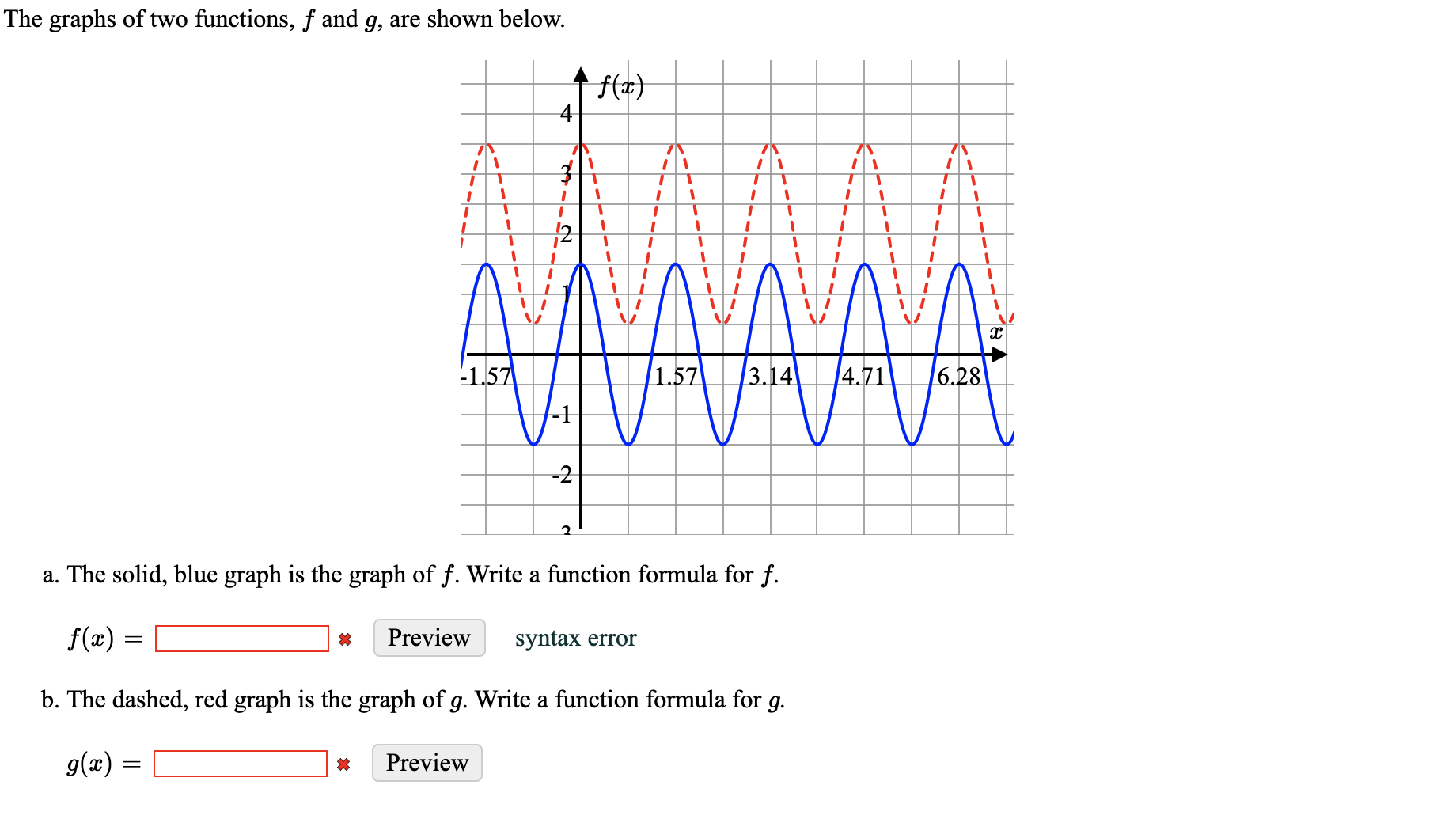# The graphs of two functions, f and g, are shown below.f(x)тт3DAAA-1.57|1.573.144.716.28-1-2a. The solid, blue graph is the graph of f. Write a function formula for f.f(x) :Previewsyntax errorb. The dashed, red graph is the graph of g. Write a function formula for g.g(x) =Preview

Question
12 viewshelp_outlineImage TranscriptioncloseThe graphs of two functions, f and g, are shown below. f(x) т т 3D AAA -1.57 |1.57 3.14 4.71 6.28 -1 -2 a. The solid, blue graph is the graph of f. Write a function formula for f. f(x) : Preview syntax error b. The dashed, red graph is the graph of g. Write a function formula for g. g(x) = Preview fullscreen
check_circle

Step 1

Period of the blue graph is 1.57

It has max=1.5 and min =-1.5

So, amplitude...

### Want to see the full answer?

See Solution

#### Want to see this answer and more?

Solutions are written by subject experts who are available 24/7. Questions are typically answered within 1 hour.*

See Solution
*Response times may vary by subject and question.
Tagged in